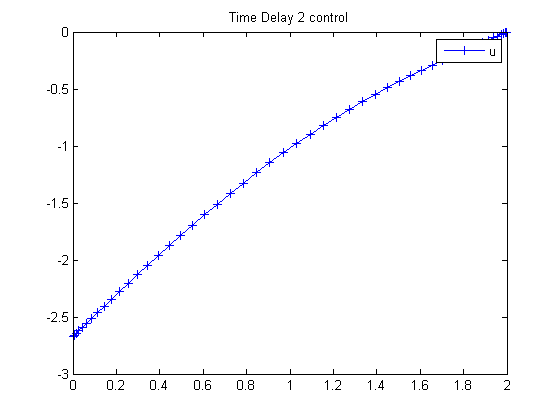116  Time Delay 2 (Approximate)

ITERATIVE DYNAMIC PROGRAMMING, REIN LUUS

8.3.2 Example 2

CHAPMAN & HALL/CRC Monographs and Surveys in Pure and Applied Mathematics

Linear time-delay system considered by Palanisamy et al.

116.1  Problem Formulation

Find u over t in [0; 2 ] to minimize

 J = x2(tF)

subject to:

 dx1 dt
= t*x1 + x1(ttau) + u
 dx2 dt
= x12 + u2
 tau = 1

A Taylor series expansion gives:

 dx1 dt
≈
 (t+1)*x1 + u 1 + tau

The initial condition are:

 x(0) = [1  0]
 −inf <= u <= inf

Reference: 

116.2  Problem setup

toms t
p = tomPhase('p', t, 0, 2, 50);
setPhase(p);

tomStates x1 x2
tomControls u

% Initial guess
x0 = {icollocate({x1 == 1; x2 == 0})
collocate(u == 0)};

% Boundary constraints
cbnd = initial({x1 == 1; x2 == 0});

% ODEs and path constraints
tau = 1;
ceq = collocate({
dot(x1) == ((t+1).*x1+u)/(1+tau)
dot(x2) == x1.^2+u.^2});

% Objective
objective = final(x2);

116.3  Solve the problem

options = struct;
options.name = 'Time Delay 2 Appr.';
solution = ezsolve(objective, {cbnd, ceq}, x0, options);
t = subs(collocate(t),solution);
u = subs(collocate(u),solution);
Problem type appears to be: lpcon
Starting numeric solver
===== * * * =================================================================== * * *
TOMLAB - Tomlab Optimization Inc. Development license  999001. Valid to 2011-02-05
=====================================================================================
Problem: ---  1: Time Delay 2 Appr.             f_k       5.340734691399960700
sum(|constr|)      0.000000236393505091
f(x_k) + sum(|constr|)      5.340734927793465500
f(x_0)      0.000000000000000000

Solver: snopt.  EXIT=0.  INFORM=1.
SNOPT 7.2-5 NLP code
Optimality conditions satisfied

FuncEv    1 ConstrEv   31 ConJacEv   31 Iter   26 MinorIter  138
CPU time: 0.218750 sec. Elapsed time: 0.218000 sec.

116.4  Plot result

figure(1)
plot(t,u,'+-');
legend('u');
title('Time Delay 2 control');